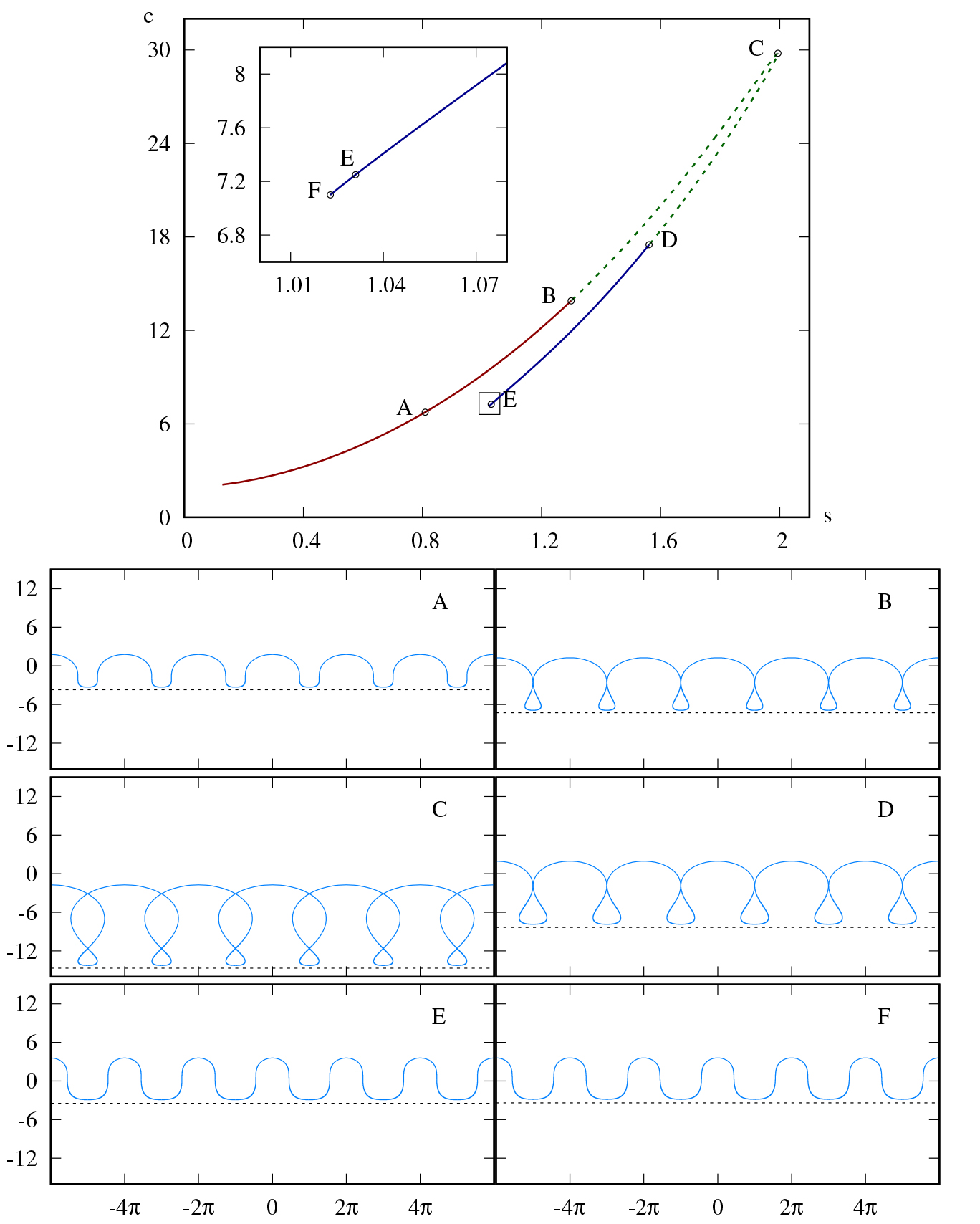### Research Highlights#### Mathematical Optimization of Systems Impacted by Rare, High-Impact Random Events

ICERM - August 2019

Decision-making in systems impacted by rare, high-impact uncertain events is important and challenging due to the potential for extreme adverse effects. Mathematical optimization is a powerful tool that can help decision-makers design, plan, and operate complex systems, but planning for the possibility of such events introduces significant mathematical modeling and computational challenges. To...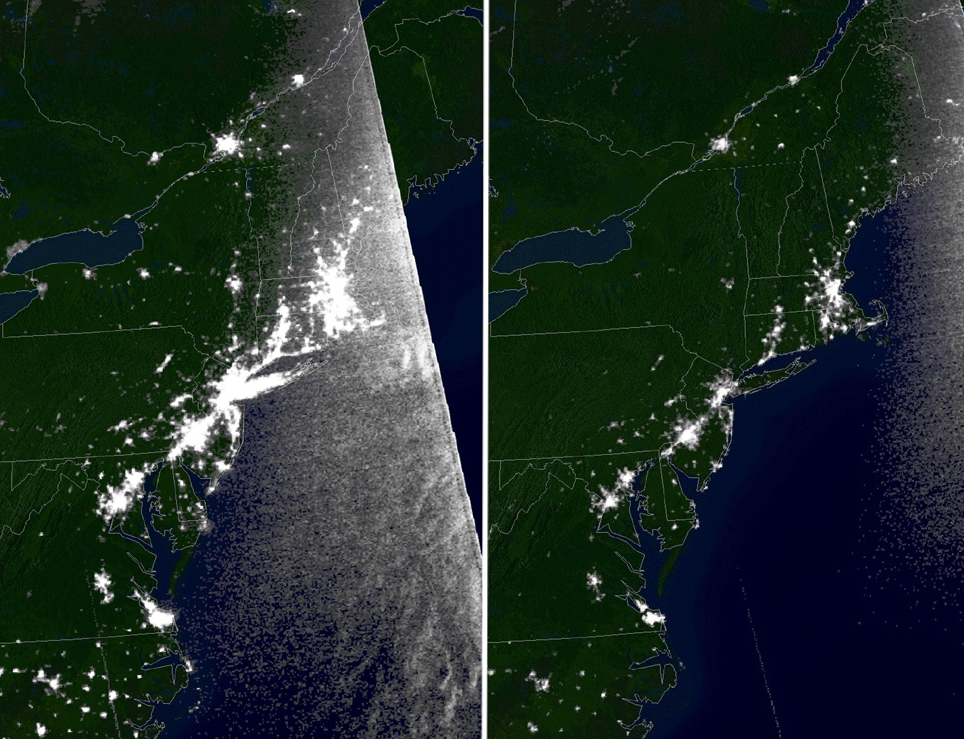#### Magnetic Bottles for Fusion Energy

MSRI - July 2019

A plasma is like a square dance gone awry. As the pace and intensity of the music (the temperature) increases, the partners (the atoms) separate into unattached dancers (electrons and ions) interacting throughout a riotous turbulent sea of independent participants (a plasma). The study of hot plasmas is central to the search for the limitless supply of energy by the nuclear fusion reaction and...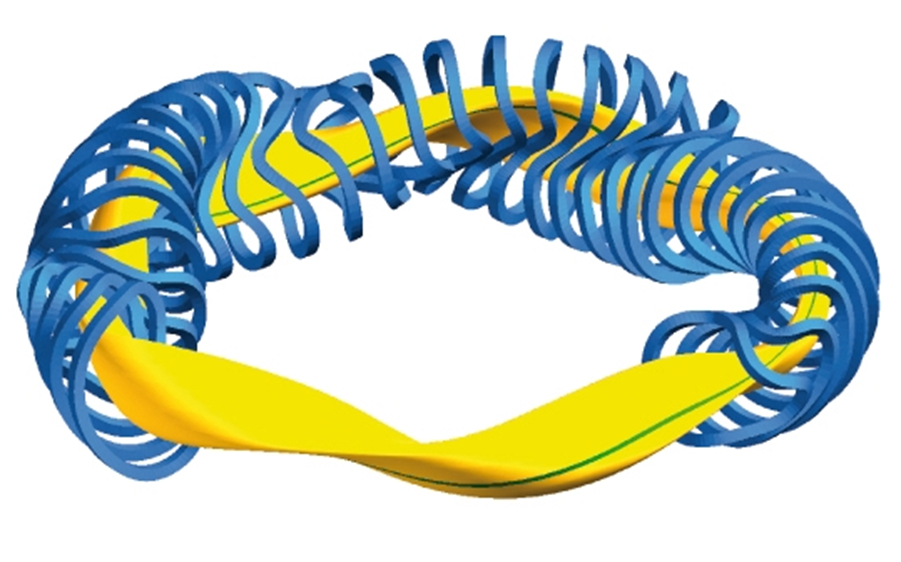#### Varieties of Varieties

MSRI - July 2019

Polynomials are fundamental mathematical objects. Many basic practices in our lives are expressed through polynomials, and the same is true for the laws of science. Einstein’s famous formula expresses energy, E, in terms of a polynomial of degree 1 in m – the mass – and of degree 2 in c – the speed of light, as E = mc2. Algebraic geometry studies those geometric objects, called (algebraic)...#### Projective geometry of Wachspress coordinates

ICERM - April 2019

The barycentric coordinates of a triangle were introduced by August F. Möbius in 1827. They describe a point in a triangle relative to the three vertices of the triangle. A point in the interior of a triangle splits the triangle into three smaller triangles, as seen in the first figure. The ratios of the three areas of the smaller triangles by the area of the big triangle are the barycentric...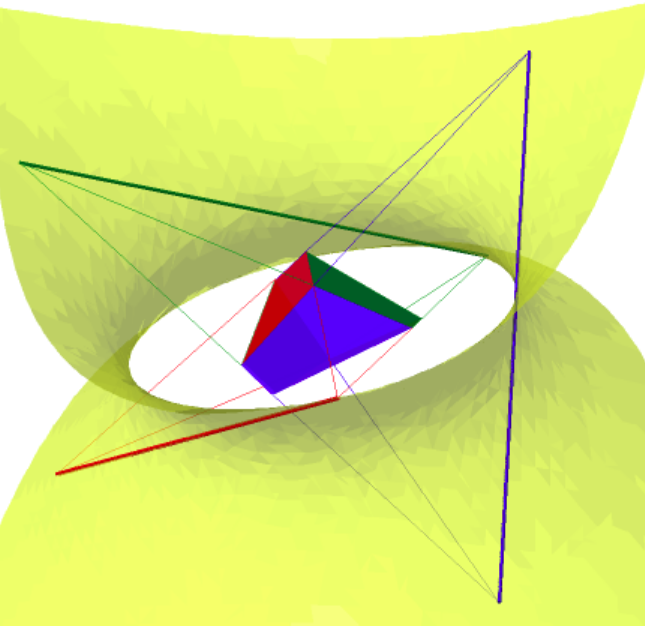#### Cutting Cakes and Splitting Rent – Combinatorics and Fair Division

MSRI - January 2019

How do you divide a cake fairly among several people with different preferences? How do you assign rooms and divide the rent among roommates so that no one envies the room and portion of rent paid by the other? Both of these are questions of fair-division, and mathematicians have shown that answers can be found using a combinatorial result known as Sperner’s Lemma. Sperner’s Lemma says the...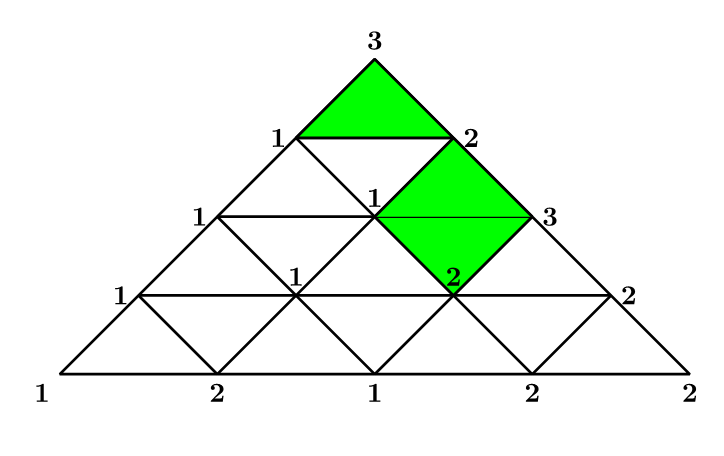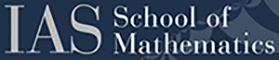#### IAS Special Year 2017-2018: Analysis and Topology on Locally Symmetric Spaces

IAS - December 2018

The subject of the special year was locally symmetric spaces. Examples of these highly symmetric objects are the hyperbolic geometries investigated by Bianchi in the 19th century (the first illustration (on the left) is from Bianchi’s 1893 paper) and a 6-dimensional space parameterizing all possible three-dimensional lattices up to rotation (the second illustration (on the right) shows such a...#### Minimizing P-Frame Energy

ICERM - November 2018

Figure 1. Projections of a configuration on the unit sphere in [math]\$ \mathbb{R}^6 \$[/math], consisting of the coordinate axes and vertices of the inscribed hemicube; i.e., the black vertices of the cube in a black and white coloring with alternating order. The 64 total vertices of the 6-dimensional cube give 32 vertices of the hemicube; adding 12 points on the axes amounts to 44 vectors....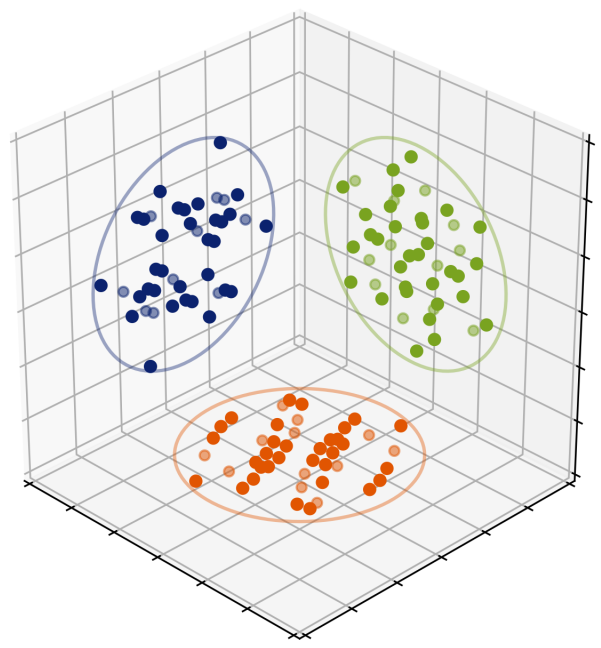#### SAMSI Blog: Where are you Gonna go when the Volcano blow?

SAMSI - October 2018

This article provided by Bruce Pitman, Professor in the Department of Materials Design and Innovation School of Engineering and Applied Science at the University of Buffalo. It highlights how the practice of statistics is used to mitigate risk hazards during natural disasters, such as a volcanic eruption. One of the major hazards of volcanic activity is inundation by debris flows, block and ash...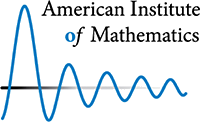AIM - October 2018

What is like to live the life of a math professor? There are many answers to this question, and they very much depend upon the nature of the mathematician’s institution. A professor at a private research university will have a different set of responsibilities and priorities to one at a liberal arts college, and different again, to someone at a large, state university. Differences also emerge...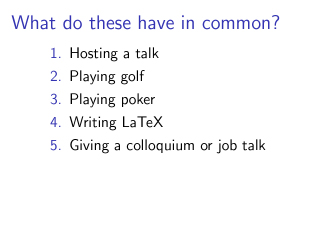#### Undergraduate Proves a Conjecture in the Theory of Water Waves

ICERM - September 2018

Seung Wook So, an undergraduate participant in ICERM’s Spring 2017 program on “Singularities and Waves In Incompressible Fluids,” discovered a proof of a conjecture in the theory of water waves. The question of how steep traveling water waves can become has a long history, going back at least to Stokes [Sto47]. During the ICERM program, So studied the specific question: how steep can traveling...